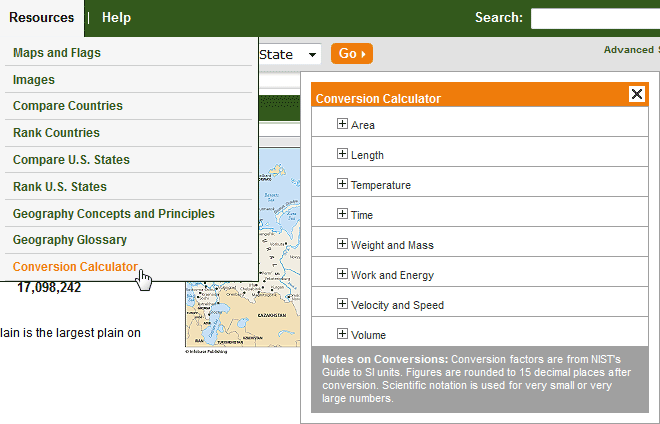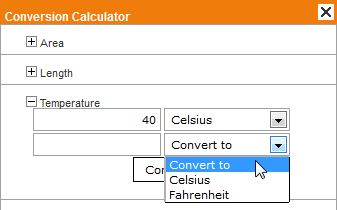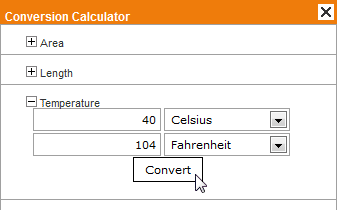# Using the Conversion Calculator

The Conversion Calculator lets you quickly convert numerical values from one measurement unit to another. Listed below are the types of measurements you can convert and the different units available for each type.

• Area - acres, square centimeters, square inches, square feet, square kilometers, square millimeters, square meters, square yards
• Length - centimeters, inches, feet, kilometers, miles, millimeters, meters, yards
• Temperature - Celsius, Fahrenheit
• Time - days , hours, minutes, seconds, years
• Velocity and Speed - centimeters/second, feet/minute, feet/second, kilometers/hour, meters/second, miles/hour
• Volume - cups, fluid ounces, gallons, liters, milliliters, pints, quarts, tablespoons, teaspoons
• Weight and Mass - grams, kilograms, metric tons, ounces, pounds, tons
• Work and Energy - Btu, calories, joules, kilowatt-hours, watts

To use the Conversion Calculator:

• Click on the Conversion Calculator link located in the top navigation bar under 'Resources'. A Conversion Calculator pop-up box will appear.1. Click on a the + or the text link for the type of measurement you want to convert. Two text boxes and drop-down menus will appear.
2. Enter your value in the top text box.
3. Choose a measurement unit to Convert from.
4. Choose a measurement unit to Convert to.
5. Click Convert.

The value for the new measurement unit will appear in the second text box.Notes on Conversions: Conversion factors are from NIST's Guide to SI units. Figures are rounded to 15 decimal places after conversion. Scientific notation is used for very small or very large numbers.

0 out of 0 found this helpful